4000-898-123

加拿大女王大学有哪些硕士专业?加拿大女王大学是加拿大*古老的大学之一，提供优秀的课程，来这里求学的人非常多，津桥留学名师今天来给大家介绍一下加拿大女王大学有哪些硕士专业，硕士专业种类很多，供大家选择的范围很广，大家可以根据自己的兴趣爱好、职业规划等来选择专业。加拿大女王大学有哪些硕士专业

女王大学位于加拿大安大略省的金斯敦，是一所中等规模的大学，拥有充满活力的社区，是雄心勃勃的人们探索激情并发挥作用的催化剂。

女王大学提供六个学术院系和学校的优秀课程：艺术与科学，工程与应用科学，商业，健康科学(医学，护理与康复治疗)，教育与法律。

 研究生课程 解剖学和细胞生物学(M / D) 历史(B / M / D)人文学科 艺术保护(M) 劳资关系(C / M) 艺术史和艺术(B / M / D) 日本语言 艺术和科学 法(B / M) 天文学和天体物理学(B / M / D) 管理(B / M / D) 生物化学(B / M / D) 数学(B / M / D) 生物学(B / M / D) 数学与工程(B / M / D) 商业(B / M / D) MBA(M) 化学工程(B / M / D) 机械工程(B / M / D) 化学(B / M / D) ) 微生物学与免疫学(M / D) 中国 采矿工程(B / M / D) 土木工程(B / M / D) 神经科学研究(M / D) 经典(B / M) 护理(B / M / D) 社区健康与流行病学(M) 职业治疗(M) 计算机工程(B / M / D) 病理学(M / D) 计算机(B / M / D) 药理学和毒理学(M / D) 文化研究(M / D) 哲学(B / M / D) 教育(B / M / D) 身体和健康教育(B / M / D) 电机工程(B / M / D) 物理科学 工程 物理治疗(M) 工程与应用科学 物理学(B / M / D) 工程化学(B / M / D) 生理学(M / D)D) 工程物理(B / M / D) 政策研究(M) 英语语言文学(B / M / D) 政治研究(B / M / D) 环境研究(B / M) 专业Mpa(M) 行政工商管理硕士(M) 心理学(B / M / D) 法国研究(B / M / D) 公共管理(M) 性别研究(B / M)M) 康复科学(M / D) 地理(B / M / D) 宗教研究(B / M) 地质工程(B / M / D) 社会科学(一般而言) 地质科学(B / M / D) 社会学(B / M / D) 德语(B / M / D) 西班牙文(B / M) 全球发展研究(B / M) 统计(B / M / D) 城市与区域规划(M) 神学(C / B / M)

女王大学吸引了来自世界各地的一些优秀学生，在一个温馨的校园内提供广泛的经验和机会，这里有114个国家的学生。国际学生约占该大学总入学人数的11%。•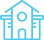国内留学规划与签证 移民一条龙服务机构
•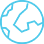中国较早聚焦专业拒 签翻案，专业移民签 证研究团队一站式服 务机构
•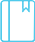合作院校众多,2600所+
•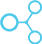在国内外多个城市设 立分支机构
•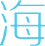在加拿大多伦多，蒙 特利尔等海外设有办 事处

worth trust International educational institution